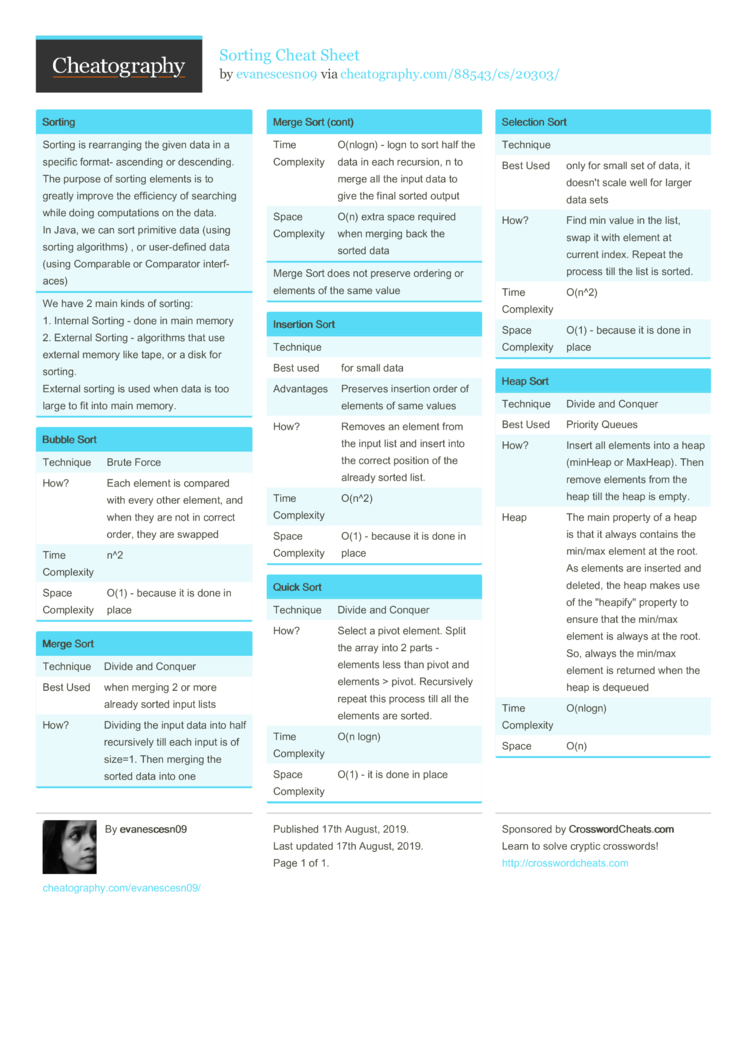# Sorting Cheat Sheet by evanescesn09

### Sorting

 Sorting is rearra­nging the given data in a specific format- ascending or descen­ding. The purpose of sorting elements is to greatly improve the efficiency of searching while doing comput­ations on the data. In Java, we can sort primitive data (using sorting algori­thms) , or user-d­efined data (using Comparable or Comparator interf­aces)
We have 2 main kinds of sorting:
1. Internal Sorting - done in main memory
2. External Sorting - algorithms that use external memory like tape, or a disk for sorting.
External sorting is used when data is too large to fit into main memory.

### Bubble Sort

 Technique Brute Force How? Each element is compared with every other element, and when they are not in correct order, they are swapped Time Complexity n^2 Space Complexity O(1) - because it is done in place

### Merge Sort

 Technique Divide and Conquer Best Used when merging 2 or more already sorted input lists How? Dividing the input data into half recurs­ively till each input is of size=1. Then merging the sorted data into one Time Complexity O(nlogn) - logn to sort half the data in each recursion, n to merge all the input data to give the final sorted output Space Complexity O(n) extra space required when merging back the sorted data
Merge Sort does not preserve ordering or elements of the same value

### Insertion Sort

 Technique Best used for small data Advantages Preserves insertion order of elements of same values How? Removes an element from the input list and insert into the correct position of the already sorted list. Time Complexity O(n^2) Space Complexity O(1) - because it is done in place

### Quick Sort

 Technique Divide and Conquer How? Select a pivot element. Split the array into 2 parts - elements less than pivot and elements > pivot. Recurs­ively repeat this process till all the elements are sorted. Time Complexity O(n logn) Space Complexity O(1) - it is done in place

### Selection Sort

 Technique Best Used only for small set of data, it doesn't scale well for larger data sets How? Find min value in the list, swap it with element at current index. Repeat the process till the list is sorted. Time Complexity O(n^2) Space Complexity O(1) - because it is done in place

### Heap Sort

 Technique Divide and Conquer Best Used Priority Queues How? Insert all elements into a heap (minHeap or MaxHeap). Then remove elements from the heap till the heap is empty. Heap The main property of a heap is that it always contains the min/max element at the root. As elements are inserted and deleted, the heap makes use of the "­hea­pif­y" property to ensure that the min/max element is always at the root. So, always the min/max element is returned when the heap is dequeued Time Complexity O(nlogn) Space O(n)1 Page
//media.cheatography.com/storage/thumb/evanescesn09_sorting.750.jpg

PDF (recommended)

Most useful cheat sheet I've seen in a long time. Add RadixSort. Its the best for special cases. Give worst-case complexity for each algorithm (and when it is to be expected. e.g. that QuickSort degrades to O(n^2) on already sorted data -- which is dangerous because it happens often in practice). You could add an introductory note on the danger of already-sorted data on some many of the algorithms. Or maybe add a section on each "complexity on pre-sorted input"?